# Mixed Numbers and Improper Fractions

Numbers come in many shapes and sizes. We are going to focus on two types.

Mixed Numbers - a whole number and a proper fraction (i.e.,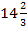)

Improper Fractions - a fraction where the top is larger than the bottom. (i.e.,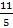)

A mixed number can be changed to an improper fraction. AND an improper fraction can be changed to a mixed number.

Let's look at the steps:

Example #1:So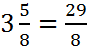.

So how might we be able to do this without the picture every time?

There were 3 wholes with 8 pieces each. So we have 3 x 8 pieces. Then there were an addition 5 pieces that we need to add on. So the numerator becomes 3 x 8 + 5. The denominator stays the same.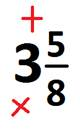In general terms, you multiple the denominator by the whole number. Then you add the numerator. This becomes the new numerator.

The denominator stays the same.

Try some more!

Example #2: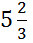=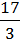Example #3: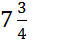You can also change from an improper fraction back to a mixed number. Take a look at the steps.

Example #4: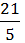Start by dividing the denominator into the numerator.

21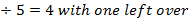The whole number part of the quotient becomes the whole number in the mixed number. The part that is left over becomes the numerator and the denominator stays the same.

21Example #5: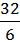Start by dividing 32 ÷ 6.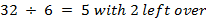.

Therefore,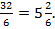We could them simplify this fraction a step further and get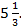Math
Fractions
Mixed number to improper fraction worksheets
Improper Fractions and Mixed number worksheets
Convert Decimals to Fractions Quiz
Add and Subtract Like Fractions Quiz
Add and Subtract unlike fractions Quiz
Multiply Fractions Quiz
Dividing Fractions Quiz
Factors